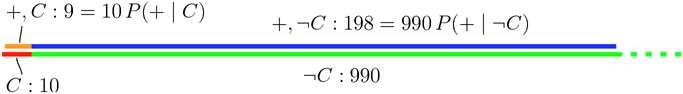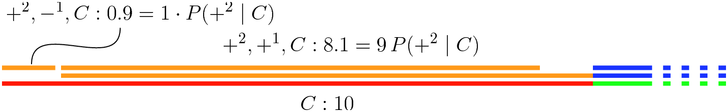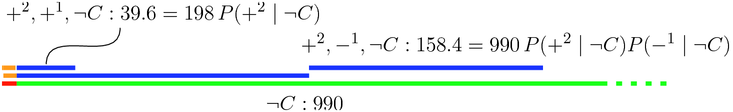## Visual Bayes

### A graphic explanation of the Bayes theorem

I enjoyed how the 3.16 section of the Stanford Artificial Intelligence class presented the Bayes theorem. Instead of giving a formula and expecting the alumni to apply it, they gave us a problem that the Bayes theorem would solve and expected, I believe, that we figured it out ourselves.

Being as I am counting-challenged, it took me a while to figure out a way of solving it that was simple enough that I could be reasonably sure of my results. It turned out to be a very interesting detour.

The problem was like this: the probability of having cancer is $P(C)=0.01$; the probability of giving positive in a cancer test when you have cancer is $P(+ \mid C)=0.9$; and the probability of giving positive when you don't have cancer is $P(+ \mid \neg C)=0.2$. What is the probability of having cancer if you give positive in the test?

It's interesting because it is sort of how it works. A test for cancer is not tried on all the population. You find some people that have cancer, give them the test, and see what proportion comes positive; you find other people that don't have cancer, give them the test, and see how many come positive as well. But that doesn't directly tell you what proportion of the people who give positive in the test will actually have cancer.

Let's assume a population of 1000:The bottom line represents the full population. The red at the left is the $0.01$ who have cancer, and the green the $0.99$ who don't. The top line is the people who give positive in the test. The orange spec at the left are those who have cancer and give positive, and the blue are those who don't have cancer but also give positive.

Zooming in:In red on the left we have the $0.01 \cdot 1000 = 10$ persons who have cancer, and on top in orange those among them who give positive in the test. We know that $P(+\mid C)=0.9$ —90% of people who have cancer give positive— so $0.9\cdot 10=9$ persons have cancer and give positive.

In blue we have the people who don't have cancer but give positive. Given that $P(+\mid \neg C)=0.2$ —20% of people who don't have cancer give positive— there will be $0.2\cdot 990=198$ healthy persons who give positive.

Now the answer to the problem should be obvious. We know that the test is positive, so that puts us in the top line. Out of the $9+198=207$ persons who give positive, only $9$ actually have cancer. So the chances of having cancer given that the test is positive are $9/207=0.043$, only around 4.3%.

An interesting follow-up question would be to figure out how accurate the test would have to be for a positive to have a 50% chance of having cancer. One way would be to reduce the percentage of false positives from $0.2$ to $9/990=0.009$ (check it out, this is not obvious from the above), almost dividing it by 20.

### How about two independent tests?

Unit 3.20 asks us to figure out the probability of having cancer when two independent test are positive, assuming that the new test has the same probabilities as the previous.

The answer is about as simple as before. Let's call a positive in the first test $+^1$ and a positive in the second test $+^2$, and draw it like this:The key here is independence. If the two tests are independent, the proportion of positives in the second test among people who gave positive in the first one will be the same as among people who gave negative. That is, if we take the 9 persons who have cancer and gave positive in the first test, $P(+^2\mid C)=0.9$ of them will be positive with the second test, or $8.1$ (and $0.9$ of the $1$ who had cancer and gave negative will be positive this time).

The same applies to the 198 who don't have cancer and gave positive in the first test. As the two tests are independent, there will be $P(+^2\mid \neg C)=0.2$ of them who are positive again, or 39.6:So we have $39.6+8.1=47.7$ people who give positive in the two tests, and $8.1$ among them have cancer, so the probability of having cancer if you give positive in two independent tests is $8.1/47.7=0.17$.

### Bayes theorem

The formulation of the Bayes theorem can be given like this: if the probability of $A$ is $P(A)$, the probability of $B$ is $P(B)$, and the probability of $B$ given $A$ is $P(B\mid A)$, then the probability of $A$ given $B$ is

$P(A\mid B) = \frac{P(B\mid A) P(A)}{P(B)}.$

If you are like me, this requires some parsing. We humans are hard-wired to understand problems involving people and relationships between people: the chances that you have cancer when you have received a positive cancer test are much easier to think about than the probability of $A$ given $B$. So let's translate this to the terms of the problem we have been thinking about:

$P(C\mid +) = \frac{P(+\mid C) P(C)}{P(+)}.$

The probability of giving positive, in terms of the absolute values that we have been using, is the total number of positives —sum of the 198 persons who give positive without having cancer and the 9 persons who give positive and have cancer— divided by the 1000 of the total population. So, spelling it out,

$P(C\mid +) = \frac{0.9\cdot 10/1000}{(9+198)/1000} = \frac{9}{9+198}.$

Which is, of course, what we should have done to start with, without bothering to convert anything to absolute values and dispensing with the 1000's. But convoluted paths are sometimes fun to follow.

The best explanation I've found of the Bayes Theorem is in Alvin W. Drake's Fundamentals of Applied Probability Theory1. Unfortunately it is out of print, but you might get hold of a second-hand copy. This is the one book that helped me understand what probability is about.

Russell and Norvig's Artificial Intelligence: A Modern Approach also has a great introduction, and many interesting examples and practical uses.

### Acknowledgements

Thanks to Utpal Sarkar for his insightful comments and his review of a draft of this article.

## Related

 Magnitude — A python library for computing with physical quantities A Unicycle on a Slope Equilibria of a Unicycle Playing with convolutions in Python

## Footnotes:

1

Disclaimer: I do get a cut from your Amazon purchase. Thank you very much for your support.

Barcelona, 2011-11-01